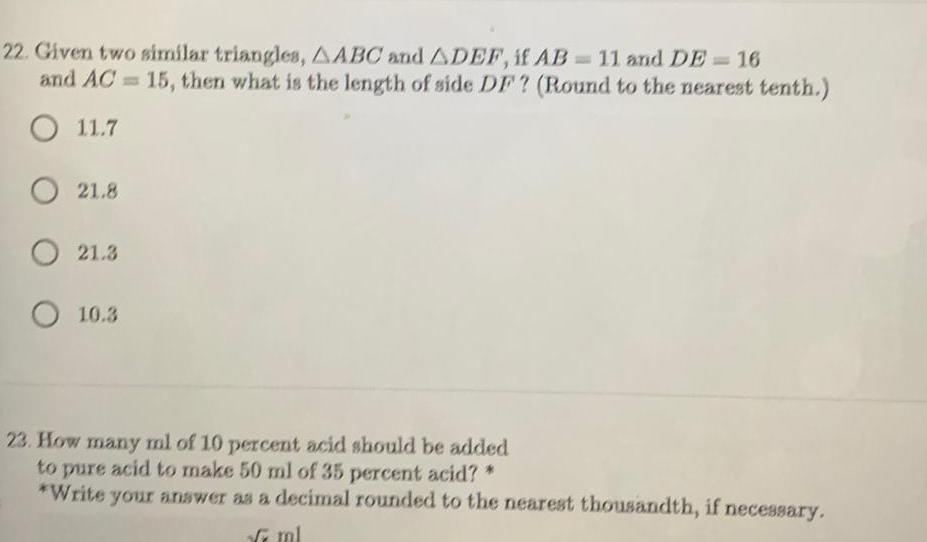Question:

# 22 Given two similar triangles AABC and ADEF if AB 11 and DE

Last updated: 9/9/202322 Given two similar triangles AABC and ADEF if AB 11 and DE 16 and AC 15 then what is the length of side DF Round to the nearest tenth O 11 7 O 21 8 O 21 3 O 10 3 23 How many ml of 10 percent acid should be added to pure acid to make 50 ml of 35 percent acid Write your answer as a decimal rounded to the nearest thousandth if necessary ml